## Overview

The goal of this exploratory analysis is to summarize basic information about the data used to create an NLP prediction algorithm and Shiny app. The exploratory analysis: 1) demostrates that the data sets are successfully downloaded and loaded into R, 2) provides relevant descriptive statistics, graphs, 3) spells out interesting observations, and 4) maps out further plans for creating a prediction algorithm and Shiny app.

The exploratory analysis is based on the English version of the Capstone Dataset. All code below assumes that the three data files, “en_US.blogs.txt”, “en_US.news.txt” and “en_US.twitter.txt,” are present in the working directory.

As Table 1 below illustrates, the twitter data file is the smallest in size but contains the largest number of lines, most likely because of the tweet character limitations. The blog data file, on the other hand is the largest in size but with the smallest number of lines since blog entries tend to be more verbose than news entries or tweets.

File Name File Size Number of Lines Number of Words Number of Word Characters
en_US.blogs.txt 210.2 MB 899288 37570839 162464653
en_US.news.txt 205.8 MB 1010242 34494539 162227130
en_US.twitter.txt 167.1 MB 2360148 30451170 125570778

## Converting To Corpus

The next step is to convert the data to corpus and extract a sample that can be handled efficiently in the computer’s RAM. Random samples of .5% of the content from each data file are merged together and tokenized into three working data files for different ngrams - single words, two-word combinations and three-word combinations. All of those procedures are done using the package “quanteda.” In addition, a the corpus is subjected to basic cleanup removing stopwords along with the hashtag symbol. It should be noted that the process is resource-intensive and, depending on the sample size, can take a long time.

Wordcloud charts are generated for each of the ngrams. The wordclould below plots the most frequent single words with size and color corresponding to frequency. For example, “said”, “just” and “one” are highest frequency words.

## Corpus consisting of 21347 documents, showing 3 documents.
##
##        Text Types Tokens Sentences
##  text397789    31     43         2
##   text74740    83    118         3
##  text552712    17     20         1
##
## Source:  Combination of corpuses sampleBlog + sampleNews and sampleTwitter
## Created: Sat Feb 11 19:30:19 2017
## Notes: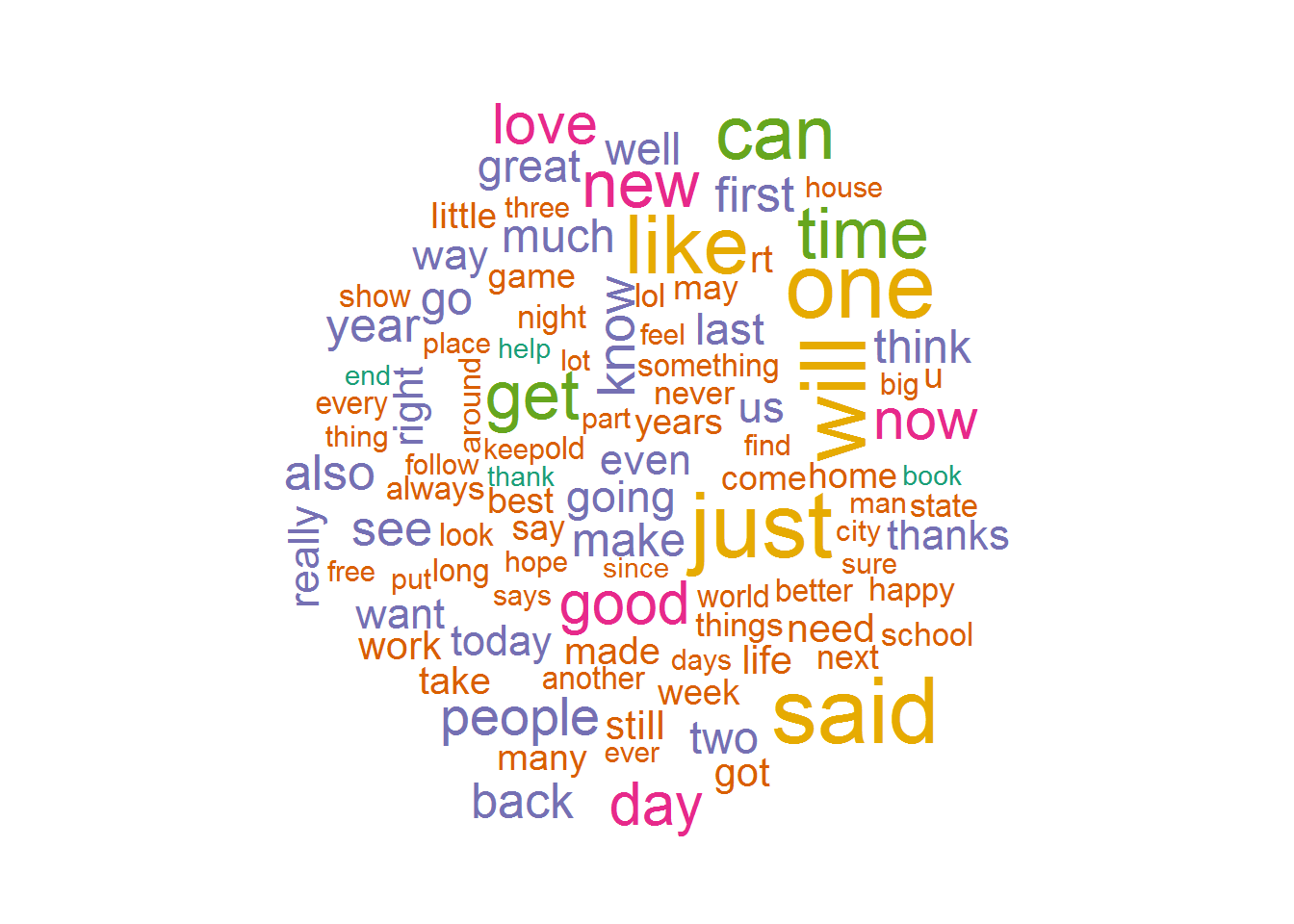The next wordcloud plots the most frequent two-word combinations. The top two-word combinations seem to be prepositions followed by “the” - “of the”, “in the”, “for the”, “on the”, “to the”.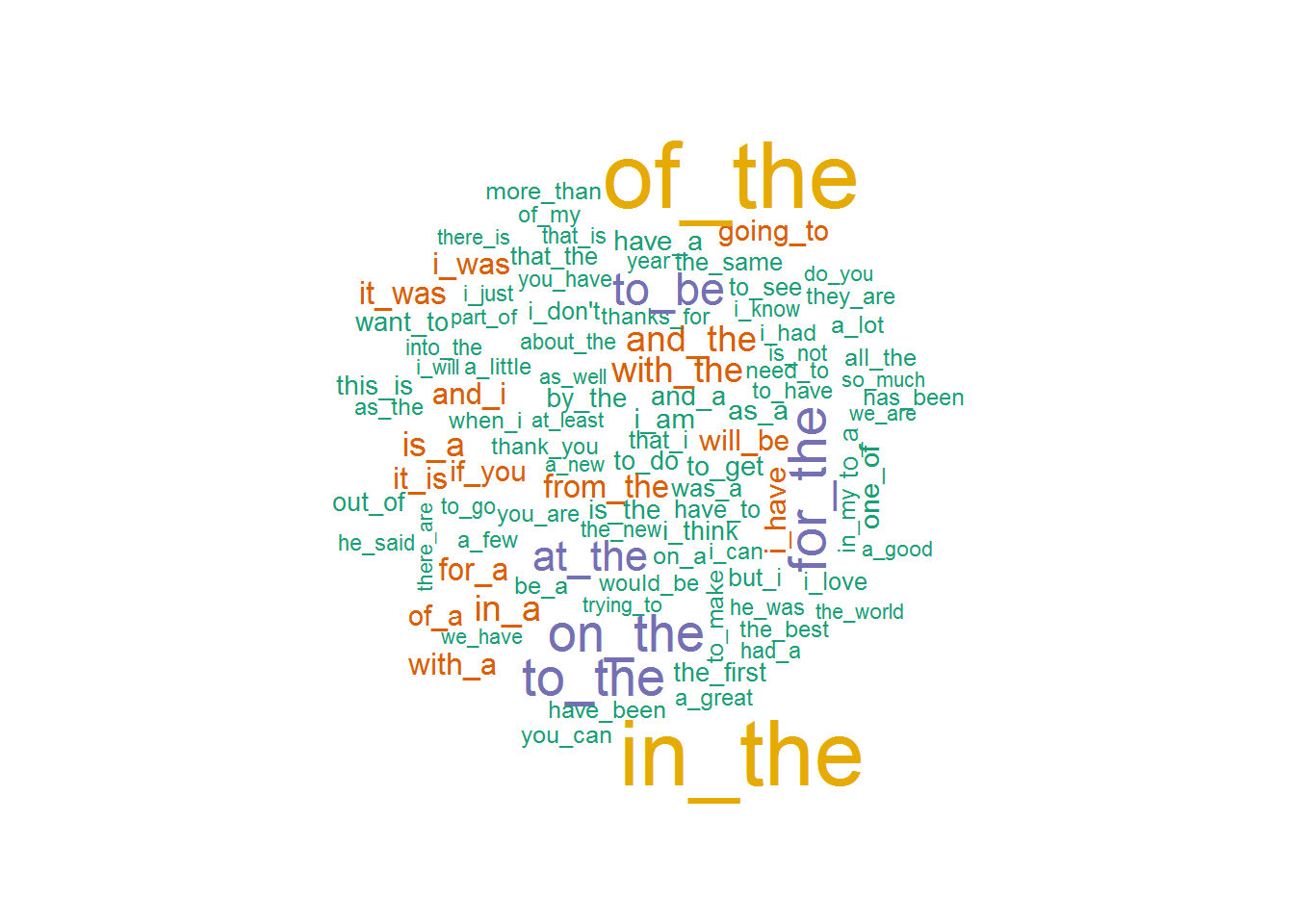The final wordcloud depicts the most frequent three-letter combinations. A number of the top combinations include the top two-letter combinations. This wordcloud contains single words, most likely a draw-back of the clean-up process. This should be taken into account during the next stages of the NLP project.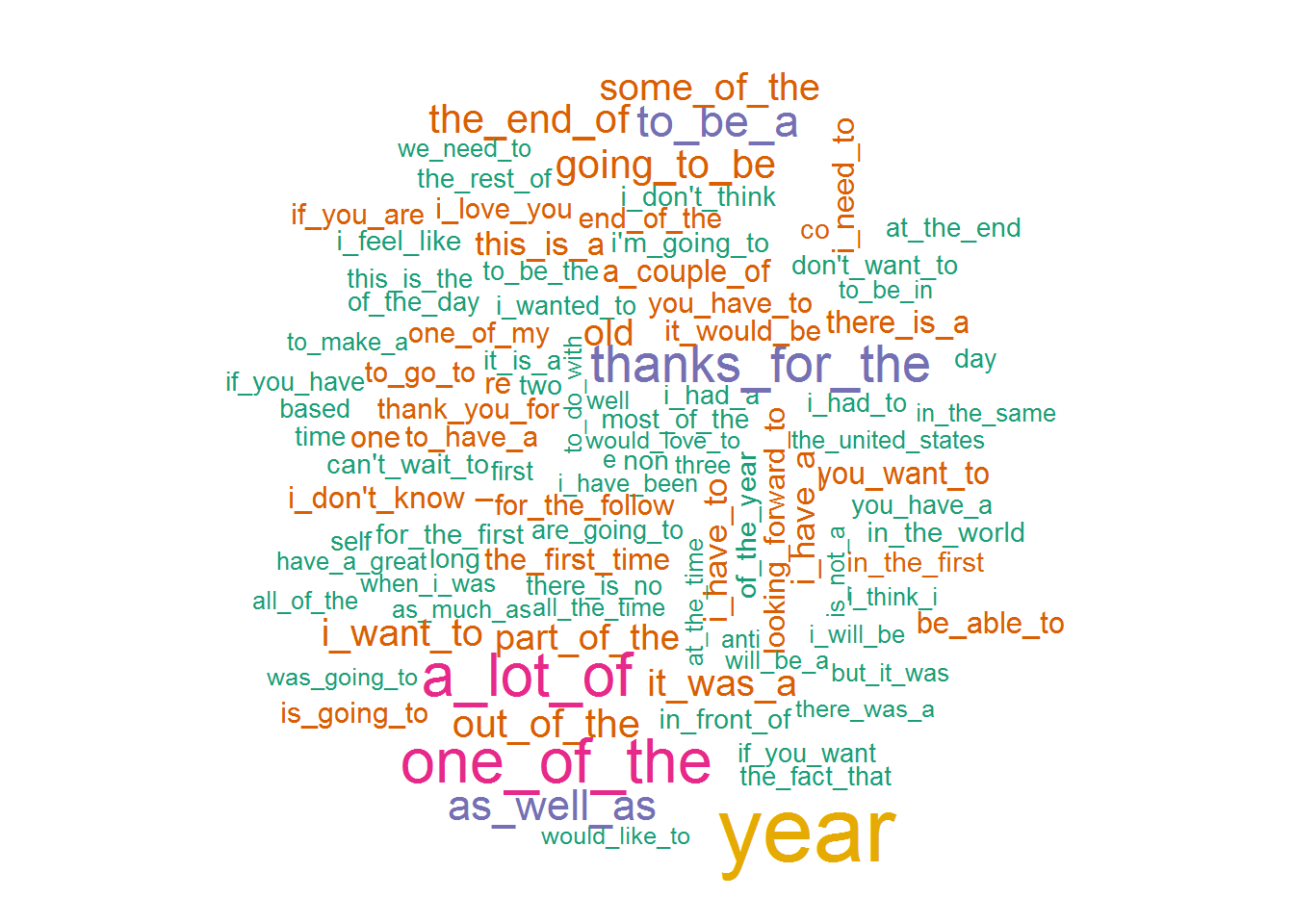Finally, the comparative frequencies of the top 30 single, two-word, and three-word combinations are plotted below. All three charts illustrate that the most frequent words and combinations are far from being evenly distributed. Specifically, the top two two-word combinations appear in about a third of all uses. The same can be said about the top three three-word combinations.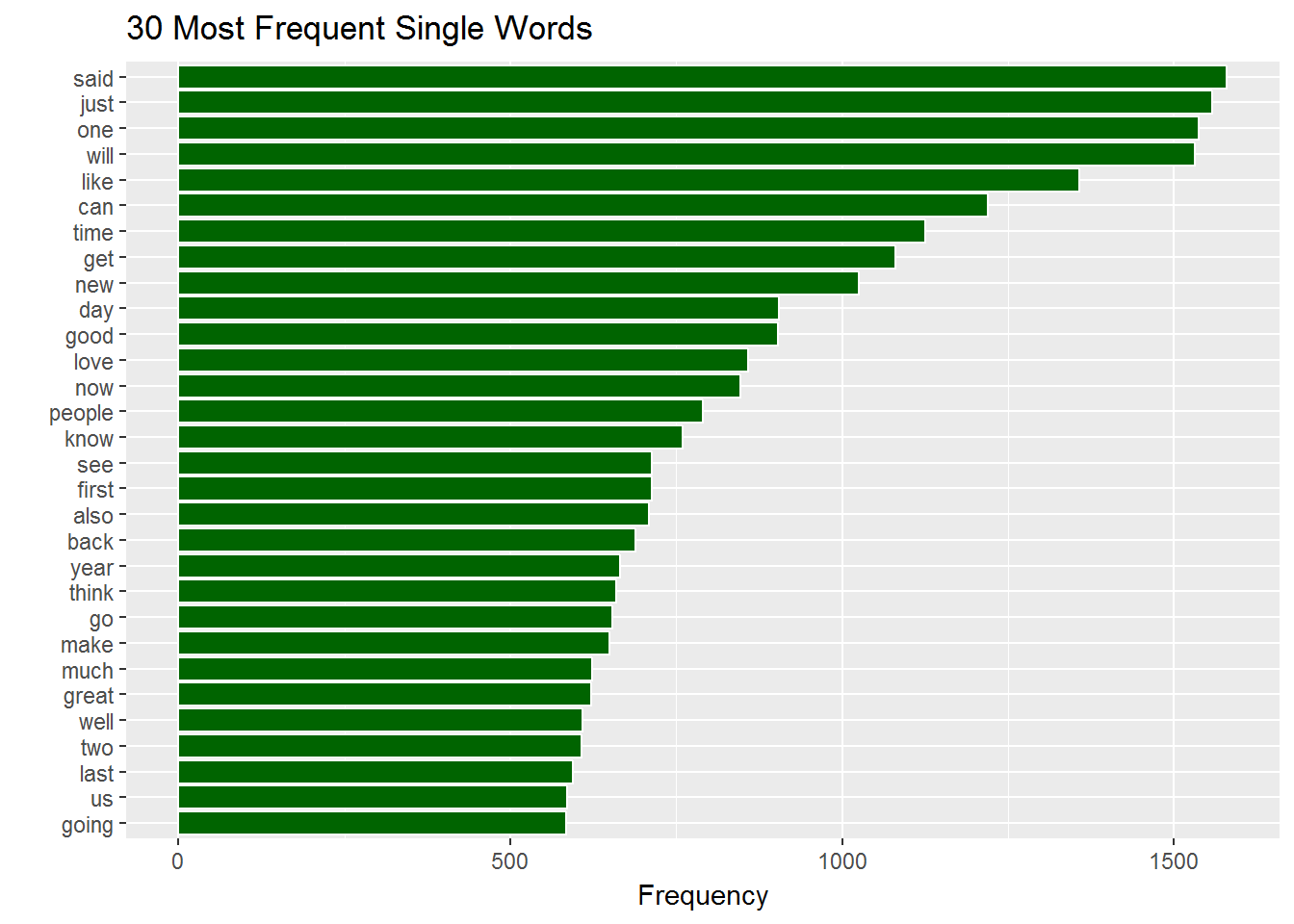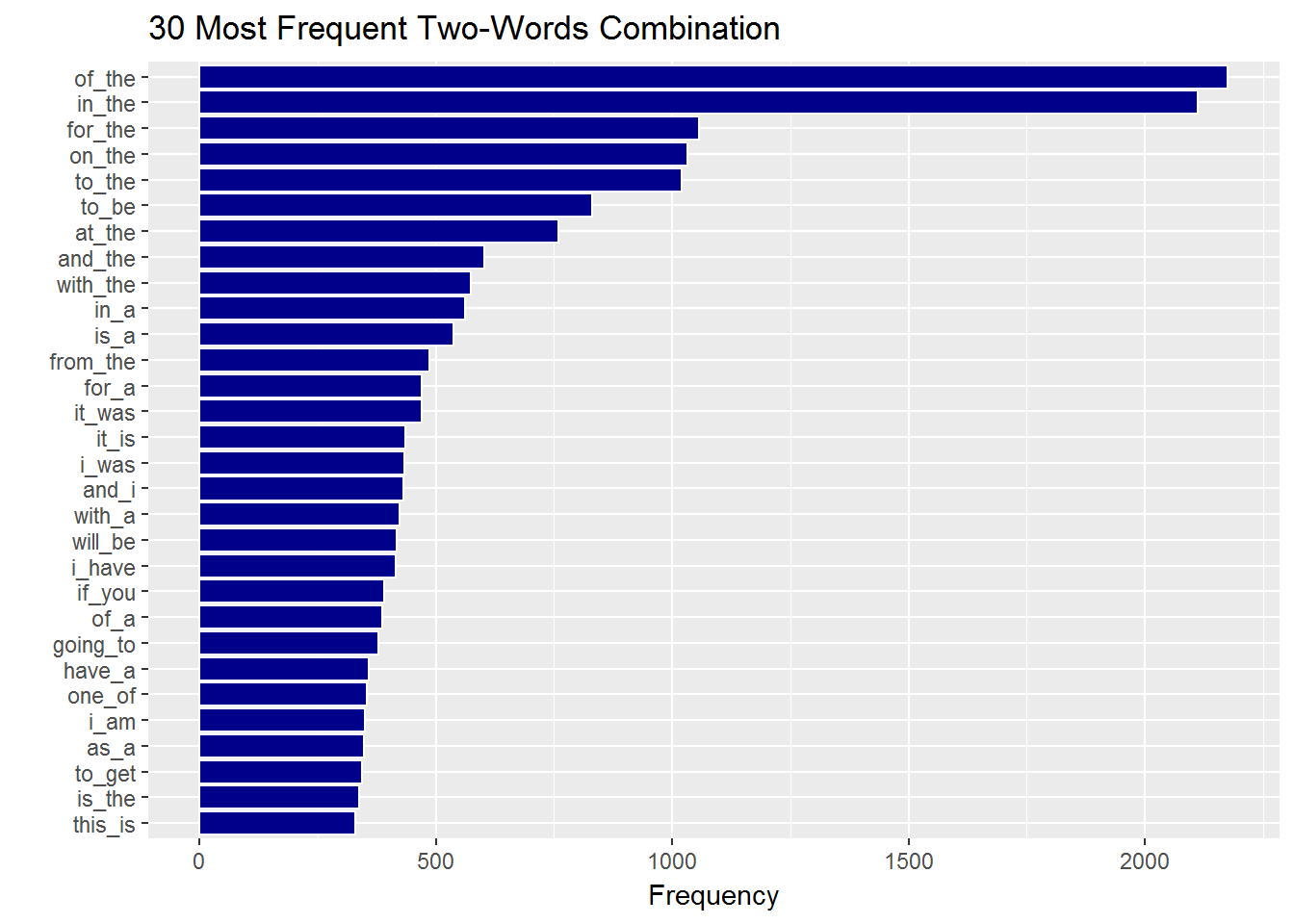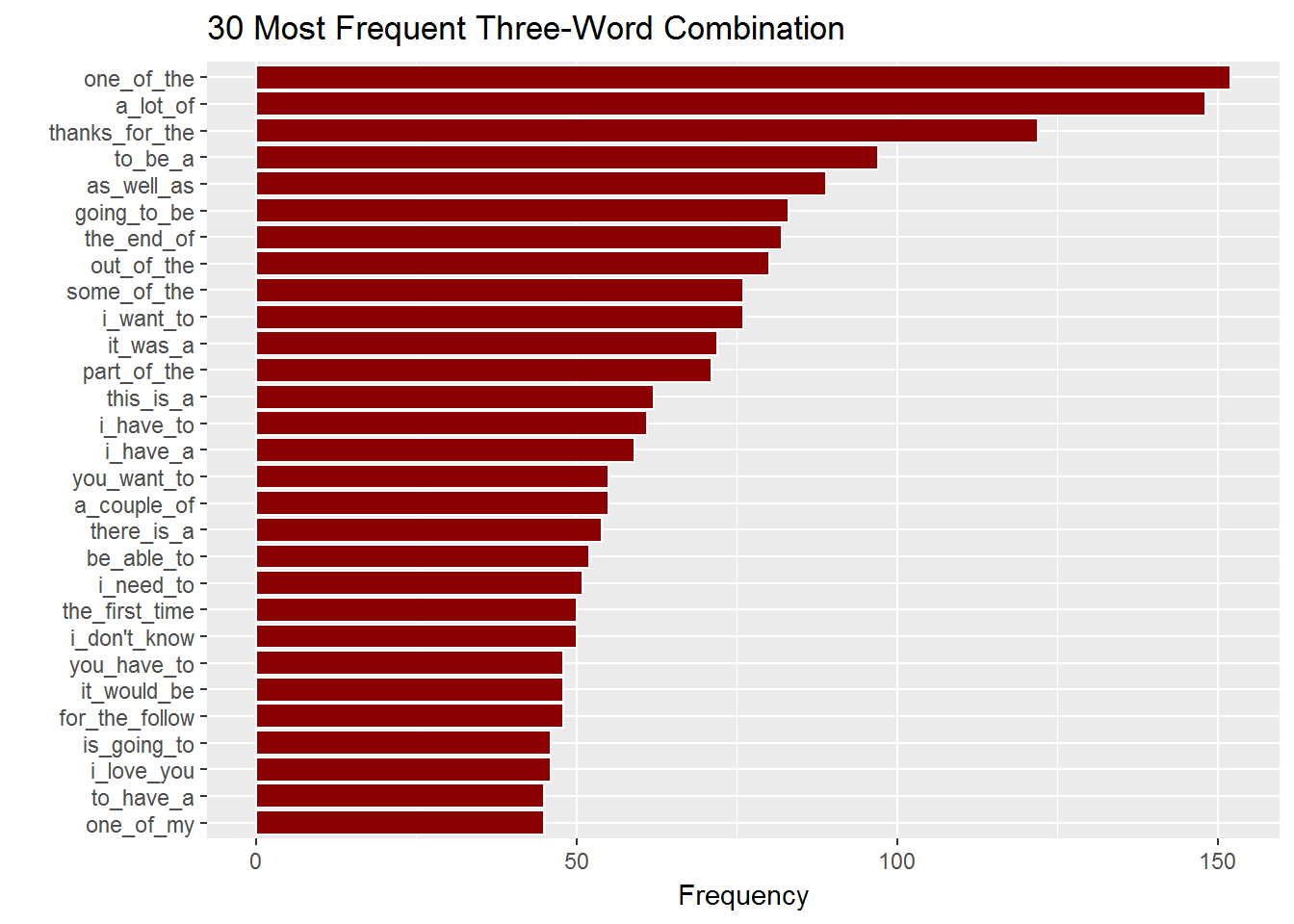## Conclusion And Next Steps

The exploratory analysis shows that data are rich and would be useful in developing a predictive model for the relationship between different words. Some relationships stand out even at this stage and can serve as the basis for back-off models. For example, if half of the top 30 bi-grams include “the”, that may be a good predictive model next step. The article may need to be removed from a sample corpus in order to consider other bi-grams and tri-grams.

The exploratory analysis also shows that there are some trade-off in terms of efficiency and accuracy. Sample sizes and clean-up procedures should be examined more carefully to develop optimal parameters for a predictive model that is both efficient and accurate. Because of the uneven distribution of the most frequent words and combinations, it is highly likely that relatively frequent instances may remain unobserved, regardless of sample sizes. If Katz’s back-off model is to be adopted, the corpus should be as large as possible.

## Appendix: Source Code

# Extract file sizes
FileStats <- as.data.frame(file.info(list.files())[1:3,1])
FileStats$FileName <- c(list.files(), list.files(), list.files()) names(FileStats) <- "FileSize" ## Convert sizes to mb library(gdata) FileStats[,1] <- humanReadable(FileStats[,1], standard="SI", units="mB") FileStats <- FileStats[c("FileName", "FileSize")] # Extract number of lines library(R.utils) FileStats$NumLines <- sapply(FileStats[,1], countLines)
# Extract number of words
blogFile <- file("en_US.blogs.txt")
newsFile <- file("en_US.news.txt")

dataBlog <- readLines(blogFile, n = -1, encoding="UTF-8", skipNul=TRUE)
close(blogFile)
dataNews <- readLines(newsFile, n = -1, encoding="UTF-8", skipNul=TRUE)
close(newsFile)

library(stringi)
wordsBlog <- stri_stats_latex(dataBlog)
wordsNews <- stri_stats_latex(dataNews)

# Add word stats to file stats
FileStats$NumWords <- c(wordsBlog, wordsNews, wordsTwitter) FileStats$NumWordChars <- c(wordsBlog, wordsNews, wordsTwitter)

# Add user friendly column names in file stats
names(FileStats) <- c("File Name", "File Size", "Number of Lines", "Number of Words",
"Number of Word Characters")
# Show table
library(knitr)
kable(FileStats)

# Converting to corpus
library(quanteda)
library(data.table)
corpusBlog <- corpus(dataBlog)
corpusNews <- corpus(dataNews)

# Setting up samples
set.seed(73)
sampleBlog <- corpus_sample(corpusBlog, FileStats[1,3]*.05)
sampleNews <- corpus_sample(corpusNews, FileStats[2,3]*.05)

# Setting up one sample data file
nlpSample <- sampleBlog + sampleNews + sampleTwitter
summary(nlpSample, 3)

# Creating sample for one-word frequencies
nlpSample1gram <- tokenize(nlpSample, removeNumber = TRUE, removePunct = TRUE, simplify = TRUE,
removeSymbols = TRUE, removeTwitter = TRUE, removeURL = TRUE)
dfmSample1gram <- dfm(nlpSample1gram, remove = c("#", "-", stopwords("english")))
dt1gram <- data.table(ngram = featnames(dfmSample1gram), count = colSums(dfmSample1gram), key = "ngram")
Sample1gramTop <- topfeatures(dfmSample1gram, n = 30, decreasing = TRUE, ci = 0.95)
if (require(RColorBrewer))
textplot_wordcloud(dfmSample1gram, max.words = 100, min.freq = 5729, colors = brewer.pal(6, "Dark2"),
scale = c(3, .3))

# Creating sample for two-word frequencies
nlpSample2gram <- tokenize(nlpSample, removeNumber = TRUE, removePunct = TRUE, simplify = TRUE,
removeSymbols = TRUE, removeTwitter = TRUE, removeURL = TRUE, ngrams = 2L)
dfmSample2gram <- dfm(nlpSample2gram, remove = c("#", "-", stopwords("english")))
dt2gram <- data.table(ngram = featnames(dfmSample2gram), count = colSums(dfmSample2gram), key = "ngram")
Sample2gramTop <- topfeatures(dfmSample2gram, n = 30, decreasing = TRUE, ci = 0.95)
if (require(RColorBrewer))
textplot_wordcloud(dfmSample2gram, max.words = 100, min.freq = 3169, colors = brewer.pal(6, "Dark2"),
scale = c(3, .3))

# Creating sample for three-word frequencies
nlpSample3gram <- tokenize(nlpSample, removeNumber = TRUE, removePunct = TRUE, simplify = TRUE,
removeSymbols = TRUE, removeTwitter = TRUE, removeURL = TRUE, ngrams = 3L)
dfmSample3gram <- dfm(nlpSample3gram, remove = c("#", "-", stopwords("english")))
dt3gram <- data.table(ngram = featnames(dfmSample3gram), count = colSums(dfmSample3gram), key = "ngram")
Sample3gramTop <- topfeatures(dfmSample3gram, n = 34, decreasing = TRUE, ci = 0.95)
if (require(RColorBrewer))
textplot_wordcloud(dfmSample3gram, max.words = 100, min.freq = 485, colors = brewer.pal(6, "Dark2"),
scale = c(3, .3))

# Plotting the frequencies
library(ggplot2)
df1 <- as.data.frame(Sample1gramTop)
df1$Word <- row.names(df1) names(df1) <- "Frequency" ggplot(df1, aes(x=reorder(Word, Frequency), y=Frequency)) + geom_bar(stat = "identity", col = "white", fill = "darkgreen") + coord_flip() + labs(title="30 Most Frequent Single Words", x="", y="Frequency") df2 <- as.data.frame(Sample2gramTop) df2$Word <- row.names(df2)
names(df2) <- "Frequency"
ggplot(df2, aes(x=reorder(Word, Frequency), y=Frequency)) +
geom_bar(stat = "identity", col = "white", fill = "darkblue") +
coord_flip() + labs(title="30 Most Frequent Two-Words Combination", x="", y="Frequency")

df3 <- as.data.frame(Sample3gramTop)
df3\$Word <- row.names(df3)
names(df3) <- "Frequency"

# Remove known single words from three-word chart
library(dplyr)
df3 <- filter(df3, Word != "year")
df3 <- filter(df3, Word != "non")
df3 <- filter(df3, Word != "old")
df3 <- filter(df3, Word != "_")
df3 <- filter(df3, Word != "re")
df3 <- filter(df3, Word != "one")
df3 <- filter(df3, Word != "self")
ggplot(df3, aes(x=reorder(Word, Frequency), y=Frequency)) +
geom_bar(stat = "identity", col = "white", fill = "darkred") +
coord_flip() + labs(title="30 Most Frequent Three-Word Combination", x="", y="Frequency")